MCAT Physical : Optics

Example Questions

1 2 4 Next →

Example Question #2 : Snell's Law

An incandescent light bulb is shown through a glass prism. The certain wavlength of the light is then directed into a glass cuvette containing an unknown concentration of protein. Commonly, this process is called spectroscopy and is used to determine the concentrations of DNA, RNA, and proteins in solutions. The indices of reflection of air, glass, and the solution are 1, 1.5, and 1.3, respectively.

At what angle would the light passing through the glass cuvette need to hit the solution inside the cuvette for no light to enter the solution?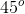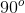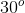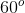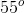Explanation:

First, we need to determine what this question is asking us to do. If no light entered the solution from the glass, we know this is total internal reflection. Remember that total internal reflection occurs when light from one medium hits a second medium at an angle higher that the critical angle. Thinking back to our physics formulas, we know that the critical angle can be determined by the equation below.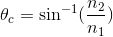Plugging in the values for glass and the solution, we can find the critical angle.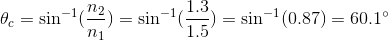Example Question #211 : Mcat Physical Sciences

A scuba diver wearing a head lamp is returning to the surface of the Pacific Ocean. What is the index of refraction of the ocean water if the smallest angle resulting in total internal reflection is 35 degrees from the vertical?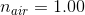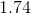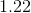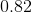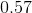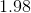Explanation:

We can use Snell's law to calculate the index of refraction of the water: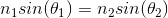Where the subscript 1 denotes water and subscript 2 denotes air.

At the first incidence of total internal reflection, the angle of refraction is 90 degrees. Therefore, the sine function becomes 1, giving us the formula: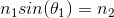Rearranging for the index of water: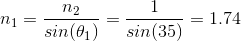1 2 4 Next →

All MCAT Physical Resources